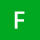Home Communities
IT Knowledge
Inspiration
Languages
EN

# Python - convert string to character array

0 points
Created by:Faith-W
503

In this article, we would like to show you how to convert string to character array in Python.

Quick solution:

``````text = 'ABC'

result = list(text)

print(result)  # ['A', 'B', 'C']``````

## 1. Practical example using `list()`

In this example, we simply use `list()` to convert `text` string to the character array.

``````text = 'ABC'

result = list(text)

print(result)  # ['A', 'B', 'C']``````

Output:

``['A', 'B', 'C']``

## 2. Alternative solution

In this example, we present how to convert `text` string to the character array without using `list()`.

``````text = 'ABC'

print([x for x in text])  # ['A', 'B', 'C']``````

Output:

``['A', 'B', 'C']``
Join to our subscribers to be up to date with content, news and offers.